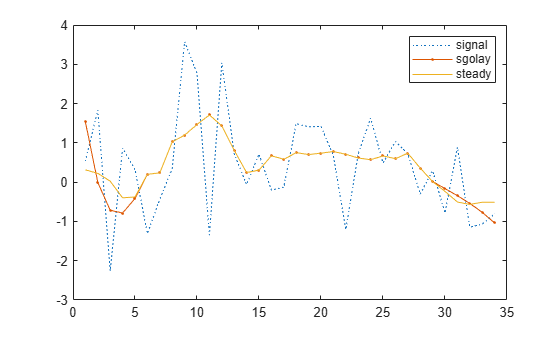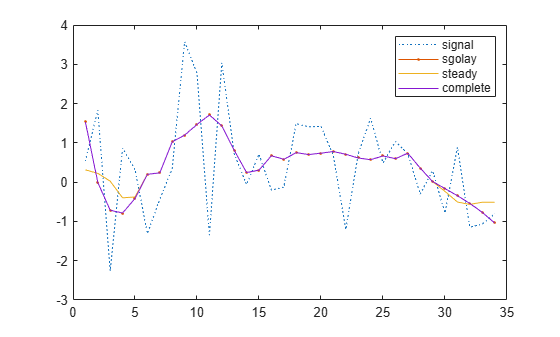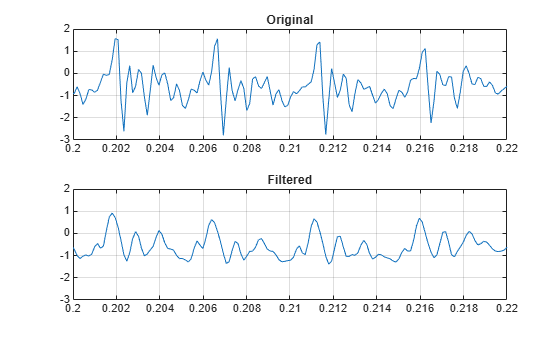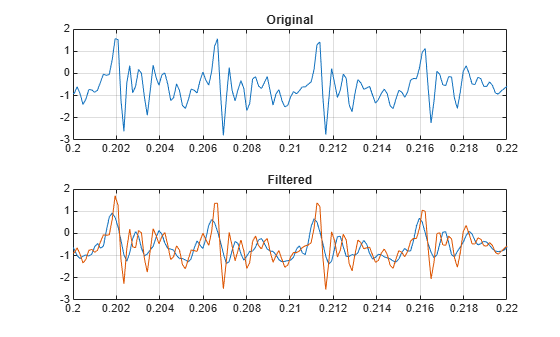# sgolayfilt

Savitzky-Golay filtering

## Syntax

``y = sgolayfilt(x,order,framelen)``
``y = sgolayfilt(x,order,framelen,weights)``
``y = sgolayfilt(x,order,framelen,weights,dim)``

## Description

example

````y = sgolayfilt(x,order,framelen)` applies a Savitzky-Golay finite impulse response (FIR) smoothing filter of polynomial order `order` and frame length `framelen` to the data in vector `x`. If `x` is a matrix, then `sgolayfilt` operates on each column. ```

example

````y = sgolayfilt(x,order,framelen,weights)` specifies a weighting vector to use during the least-squares minimization.```
````y = sgolayfilt(x,order,framelen,weights,dim)` specifies the dimension along which the filter operates.```

## Examples

collapse all

Generate a random signal and smooth it using `sgolayfilt`. Specify a polynomial order of 3 and a frame length of 11. Plot the original and smoothed signals.

```order = 3; framelen = 11; lx = 34; x = randn(lx,1); sgf = sgolayfilt(x,order,framelen); plot(x,':') hold on plot(sgf,'.-') legend('signal','sgolay')```The `sgolayfilt` function performs most of the filtering by convolving the signal with the center row of `B`, the output of `sgolay`. The result is the steady-state portion of the filtered signal. Generate and plot this portion.

```m = (framelen-1)/2; B = sgolay(order,framelen); steady = conv(x,B(m+1,:),'same'); plot(steady) legend('signal','sgolay','steady')```Samples close to the signal edges cannot be placed at the center of a symmetric window and have to be treated differently.

To determine the startup transient, matrix multiply the first `(framelen-1)/2` rows of `B` by the first `framelen` samples of the signal.

`ybeg = B(1:m,:)*x(1:framelen);`

To determine the terminal transient, matrix multiply the final `(framelen-1)/2` rows of `B` by the final `framelen` samples of the signal.

`yend = B(framelen-m+1:framelen,:)*x(lx-framelen+1:lx);`

Concatenate the transients and the steady-state portion to generate the complete signal.

```cmplt = steady; cmplt(1:m) = ybeg; cmplt(lx-m+1:lx) = yend; plot(cmplt) legend('signal','sgolay','steady','complete') hold off```Adding weights to the minimization breaks the symmetry of `B` and requires extra steps for a proper solution.

Load a speech signal sampled at ${\mathit{F}}_{\mathit{s}}=7418\text{\hspace{0.17em}}\mathrm{Hz}$. The file contains a recording of a female voice saying the word "MATLAB®."

```load mtlb t = (0:length(mtlb)-1)/Fs;```

Smooth the signal by applying a Savitzky-Golay filter of polynomial order 9 to data frames of length 21. Plot the original and filtered signals. Zoom in on a 0.02-second interval.

```rd = 9; fl = 21; smtlb = sgolayfilt(mtlb,rd,fl); subplot(2,1,1) plot(t,mtlb) axis([0.2 0.22 -3 2]) title('Original') grid subplot(2,1,2) plot(t,smtlb) axis([0.2 0.22 -3 2]) title('Filtered') grid```Repeat the calculation, but now use a Kaiser window as a weighting vector. Specify a shape factor $\beta =38$. Plot the new filtered signal.

```kmtlb = sgolayfilt(mtlb,rd,fl,kaiser(fl,38)); subplot(2,1,2) hold on plot(t,kmtlb) axis([0.2 0.22 -3 2]) hold off```## Input Arguments

collapse all

Input signal, specified as a vector or matrix.

Data Types: `single` | `double`

Polynomial order, specified as a positive integer. `order` must be smaller than `framelen`. If `order` = `framelen` – 1, the filter produces no smoothing.

Data Types: `single` | `double`

Frame length, specified as a positive odd integer.

Data Types: `single` | `double`

Weighting array, specified as a real positive vector or matrix of length `framelen`.

Data Types: `single` | `double`

Dimension to filter along, specified as a positive integer scalar. By default, `sgolayfilt` operates along the first dimension of `x` whose size is greater than 1.

Data Types: `single` | `double`

## Output Arguments

collapse all

Filtered signal, returned as a vector or matrix.

## Tips

Savitzky-Golay smoothing filters are typically used to "smooth out" a noisy signal whose frequency span (without noise) is large. They are also called digital smoothing polynomial filters or least-squares smoothing filters. Savitzky-Golay filters perform better in some applications than standard averaging FIR filters, which tend to filter high-frequency content along with the noise. Savitzky-Golay filters are more effective at preserving high frequency signal components but less successful at rejecting noise.

Savitzky-Golay filters are optimal in the sense that they minimize the least-squares error in fitting a polynomial to frames of noisy data. See `sgolay` for more information about the Savitzky-Golay algorithm.

 Orfanidis, Sophocles J. Introduction to Signal Processing. Englewood Cliffs, NJ: Prentice Hall, 1996.

 Schafer, Ronald. “What Is a Savitzky-Golay Filter? [Lecture Notes].” IEEE Signal Processing Magazine 28, no. 4 (July 2011): 111–17. https://doi.org/10.1109/MSP.2011.941097.